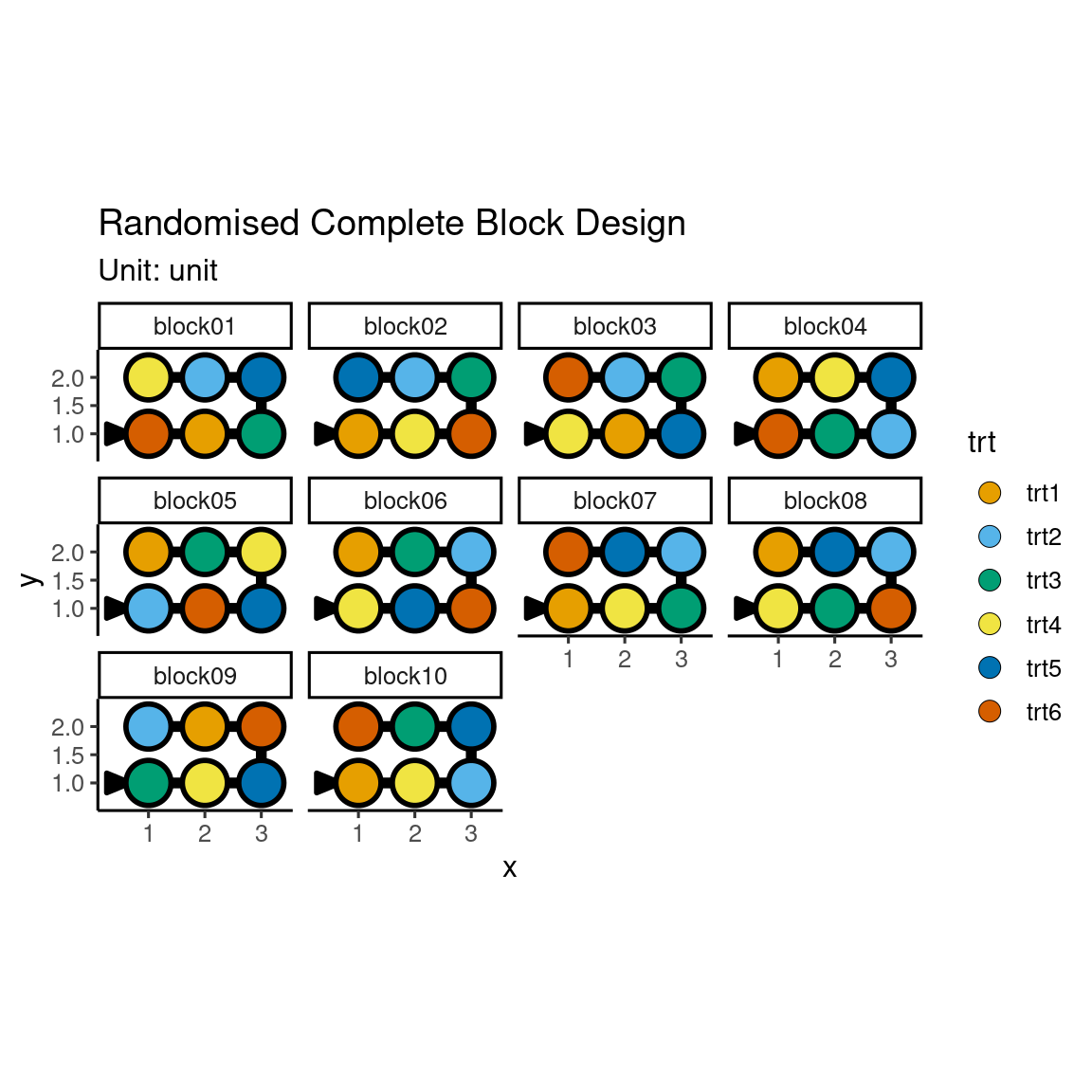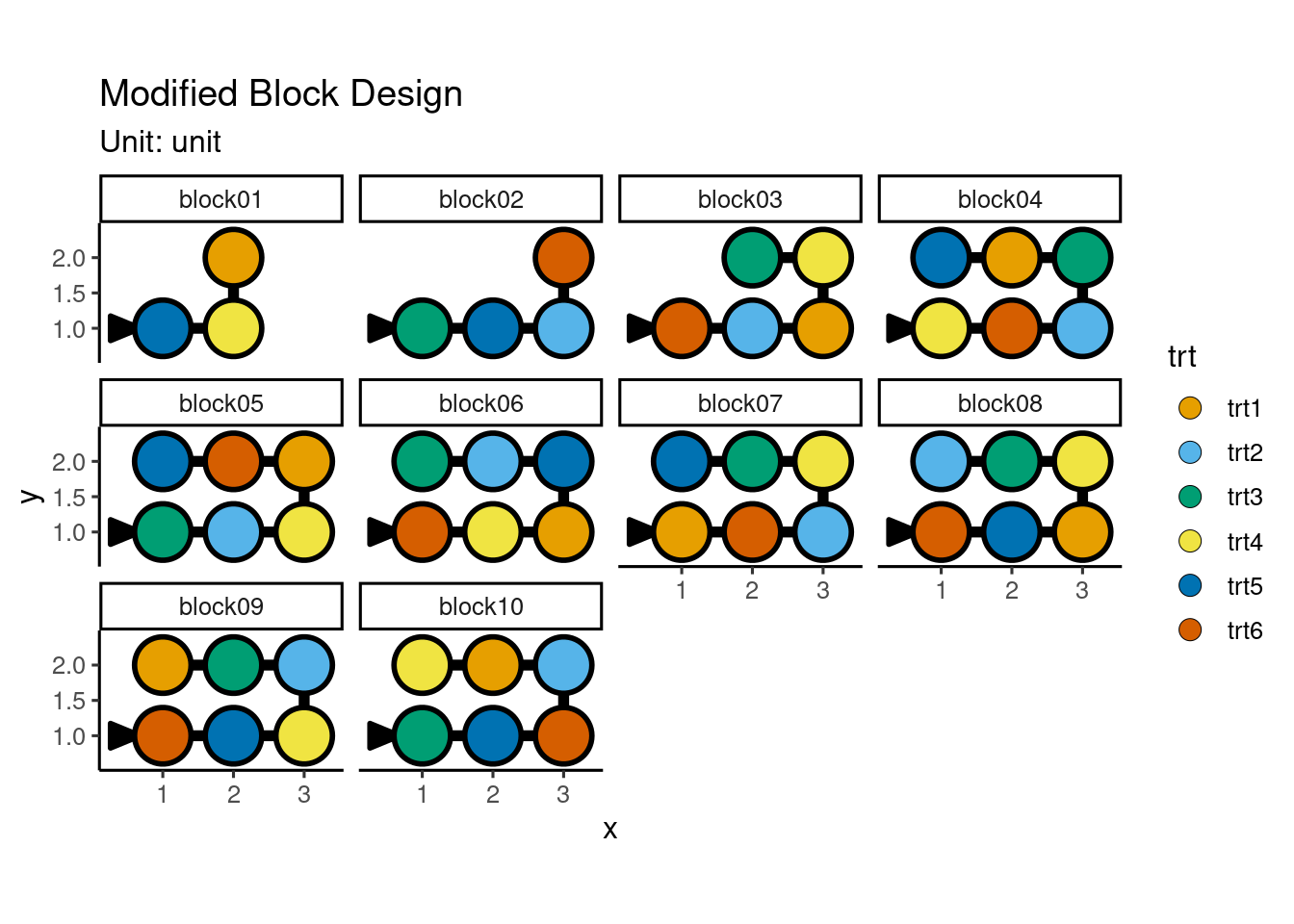📖 Status of the book

Hi there! This book is a work-in-progress. You may like to come back later when it’s closer to a complete state. If you would like to raise issues or leave feedback, please feel to do this:

A randomised complete block design, commonly abbreviated as RCBD, is contain two unit factors, a blocking factor and experimental unit factor, and a treatment factor. The experimental unit factor would be nested within the blocking factor with the number of units within each level of the blocking factor, $$k$$, equal to the number of treatments, $$t$$. Every treatment appears exactly once in each level of the blocking factor, so the number of blocks, $$b$$, is the same as the number of replications, $$r$$, i.e. $$b = r$$.

rcbd <- takeout(menu_rcbd(t = 6, r = 10))
examine_recipe(rcbd)
design("Randomised Complete Block Design") %>%
set_units(block = 10,
unit = nested_in(block, 6)) %>%
set_trts(trt = 6) %>%
allot_trts(trt ~ unit) %>%
assign_trts("random", seed = 836) %>%
serve_table()
autoplot(rcbd)Suppose now I tell you that there are only 3 units in block 1 and 4 units in block 2, and 5 units in block 3, while other blocks can have 6 units. How would you modify your design then? Still generate an RCBD but randomly remove the treatment allocation to match the block size? In comparison to the previous design, this design has 6 less units so it’s possible to have 9 replicates for each treatment. Generally you’d want to get close to a balanced design as possible so you have roughly an equal amount of information on all your treatments. If you randomly remove the treatment allocation to match the required block size, there’s no guarantee that you’ll end up with a balanced design. You’ll also find that there’ll be no named experimental design that matches the description of your units so you won’t be able to just select a design from the menu. This is where the edibble system aids you. In the fundamental edibble system, you put the experimental structure first before thinking about the treatment assignment.

mbd <- design("Modified Block Design") %>%
set_units(block = 10,
unit = nested_in(block,
1 ~ 3,
2 ~ 4,
3 ~ 5,
. ~ 6)) %>%
set_trts(trt = 6) %>%
allot_trts(trt ~ unit) %>%
assign_trts("random", seed = 326) %>%
serve_table()
autoplot(mbd)You can see below that the number of replications are equal across the treatments. You can try changing the seed number above to generate another set of design and see if it still holds true (it should!).

table(mbd\$trt)

trt1 trt2 trt3 trt4 trt5 trt6
9    9    9    9    9    9